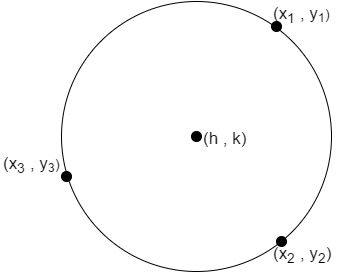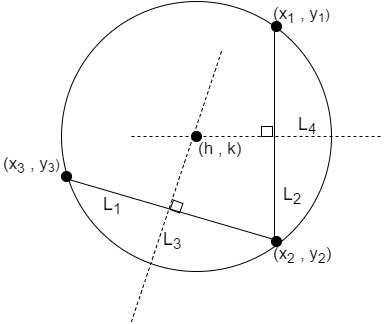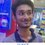# A direct relationSuppose $(x_1 , y_1)$ ,$(x_2 , y_2)$ and $(x_3 , y_3)$ are three given points on a circle. Let its center be denoted by $(h,k)$. Then,

$\boxed{h = \dfrac 12 \cdot \dfrac\sum_{(i=1,2,3) \\ (j=2,3,1) \\ (k=3,1,2)} \left( y_i - y_j \right) \left( {x_k}^2 + {y_k}^2 \right)} { \displaystyle \sum_{(i=1,2,3) \\ (j=2,3,1)} x_i y_j ~-~ \displaystyle \sum_{(i=1,2,3) \\ (j=2,3,1)} y_i x_j }$

$\boxed{k = \dfrac 12 \cdot \dfrac\sum_{(i=1,2,3) \\ (j=2,3,1) \\ (k=3,1,2)} \left( x_i - x_j \right) \left( {x_k}^2 + {y_k}^2 \right)} { \displaystyle \sum_{(i=1,2,3) \\ (j=2,3,1)} y_i x_j ~-~ \displaystyle \sum_{(i=1,2,3) \\ (j=2,3,1)} x_i y_j }}$

And the equation of circle is:

$\boxed{ {(x-h)}^2 + {(y-k)}^2 = {(h-{x_a})}^2 + {(k-{y_a})}^2 }$ where $a = 1,2,3$.

The proof is very simple yet lengthy, so I'll leave it up to the reader to find it out. You can give your proof in the comments. The diagram given below might be helpful.Note by Tapas Mazumdar
4 years, 9 months ago

This discussion board is a place to discuss our Daily Challenges and the math and science related to those challenges. Explanations are more than just a solution — they should explain the steps and thinking strategies that you used to obtain the solution. Comments should further the discussion of math and science.

When posting on Brilliant:

• Use the emojis to react to an explanation, whether you're congratulating a job well done , or just really confused .
• Ask specific questions about the challenge or the steps in somebody's explanation. Well-posed questions can add a lot to the discussion, but posting "I don't understand!" doesn't help anyone.
• Try to contribute something new to the discussion, whether it is an extension, generalization or other idea related to the challenge.

MarkdownAppears as
*italics* or _italics_ italics
**bold** or __bold__ bold
- bulleted- list
• bulleted
• list
1. numbered2. list
1. numbered
2. list
Note: you must add a full line of space before and after lists for them to show up correctly
paragraph 1paragraph 2

paragraph 1

paragraph 2

[example link](https://brilliant.org)example link
> This is a quote
This is a quote
    # I indented these lines
# 4 spaces, and now they show
# up as a code block.

print "hello world"
# I indented these lines
# 4 spaces, and now they show
# up as a code block.

print "hello world"
MathAppears as
Remember to wrap math in $$ ... $$ or $ ... $ to ensure proper formatting.
2 \times 3 $2 \times 3$
2^{34} $2^{34}$
a_{i-1} $a_{i-1}$
\frac{2}{3} $\frac{2}{3}$
\sqrt{2} $\sqrt{2}$
\sum_{i=1}^3 $\sum_{i=1}^3$
\sin \theta $\sin \theta$
\boxed{123} $\boxed{123}$

Sort by:

Is there any intuition behind the equations?

Note: It is better to write the indices as $x_i y_{i+1}$ instead of trying to express what you mean via $(i=1,2,3)(j=2,3,1)$.

Staff - 4 years, 9 months ago